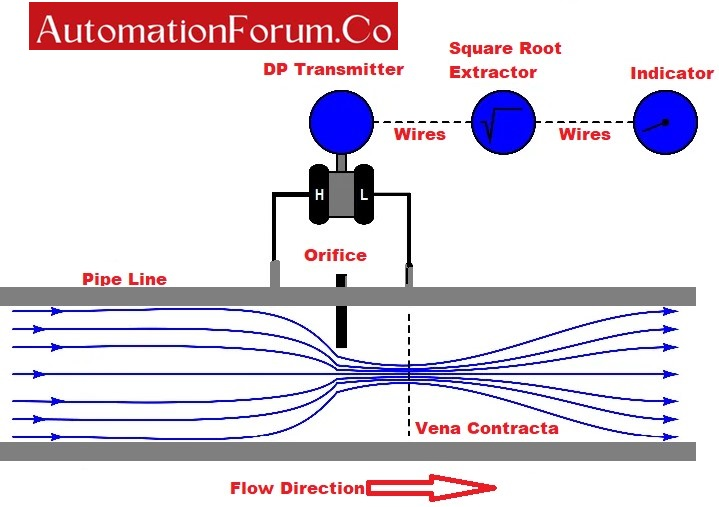# Why square root is used in flow measurement?• Differential pressure measurement is a commonly used method to measure the flow across an obstruction.
• Differential pressure is in direct proportion to the square of the flow.
• The square root relationship is applied only for DP-type flow measurements.
• These DP sensors or DP transmitters can measure differential pressure across various obstructions made in the flow channel or in a flow line.
• This differential pressure is developed by the use of primary elements such as an orifice plate, venturi tube, etc.
• But, differential pressure is not proportional to the flow rate across the orifice.
• The above relation is not a linear relationship or it is called the square root relation because the differential pressure measured has nonlinear relation with flow through the pipeline.
• The graphical relationship between differential pressure (ΔP) & flow rate (Q) is the quadratic parabola.
• A miserable significance of this quadratic relationship is that a flow rate is not directly sensed when a pressure-sensing instrument is connected to a flow sensor. But the pressure sensing instrument will sense the square of the flow rate.
• The square root function should be implemented in the loop for accurate measurement of flow throughout the operating range.
• The square root extractor converts the measured differential pressure into the rate of flow.
• Almost 50% of all flow-measuring applications use the differential pressure method to derive the flow rate. The relation between Differential Pressure (DP) and flow rate is given as

Where

Q = flow rate.

DP = differential pressure.

K = is a constant that depends on various factors such as the diameter of the pipe, orifice diameter, and so on.

# What is square root extraction in flow measurement?

• A square root extractor is a type of signal conditioner.
• It is easy to use indicators, recorders, and controllers to register linearly with the flow velocity.
• Square root extraction in flow measurement is an arithmetic conversion applied to a linear measurement scale to convert it into a nonlinear square root scale.
• The square root scale is generated by considering the square root of the ratio between measurement reading and full span.
• Square Root Extraction is sometimes called square root scaling. And it is a fundamental step in considering the flow rate from a differential pressure measurement.

# What is a square root extractor?

• A square root extractor converts a linear differential pressure signal to a nonlinear flow rate.
• The square root extractor is an electronic or pneumatic instrument that generates a corresponding linear flow signal from the flow transmitter.
• Usually, the flow transmitter signal is in the form of a square root.

# Why flow rate is directly proportional to the square root of δp?

• According to Bernoulli’s principle “the square root of DP is directly proportional flow rate. It means if the pressure is high, then the flow rate increases and vice versa.
• The flow rate in the pipe is equal to the velocity multiplied by the cross-section because the pressure comes from only one end of the pipe.
• Flow element creates a pressure change by accelerating a fluid stream in differential pressure based flow meter.
• The pipeline is intentionally narrowed to create a low-pressure region.

# Why square root is used in the flow transmitters?

Generally, the flow transmitter is configured in square root because a small change in differential pressure on the lower end results in a large change in flow.

If the square root is considered in the controller, the large change in flow makes it extra sensitive to electrical noise on the mA signal.

# Where is square root extraction performed?

• Almost modern smart differential pressure transmitters allow the DP to square root conversion to be calculated within the transmitter.
• But, all SCADA and DCS systems have inbuilt square root extraction function blocks to configure inputs from DP transmitters.
• All square root extractions are performed in one or other of these locations.
• So, the instrument and control system engineer has an option of where to have the signal conversion.

# Square root extraction formula:

A. To convert a given linear 4 to 20mA signal to a square root signal the following relation is adopted.

B. And to convert a square root signal to a linear 4 to 20mA signal use this formula:

# Square root extraction table:

The table shows values for both a linear to square root conversion and a square root to linear conversion.

Scroll to Top